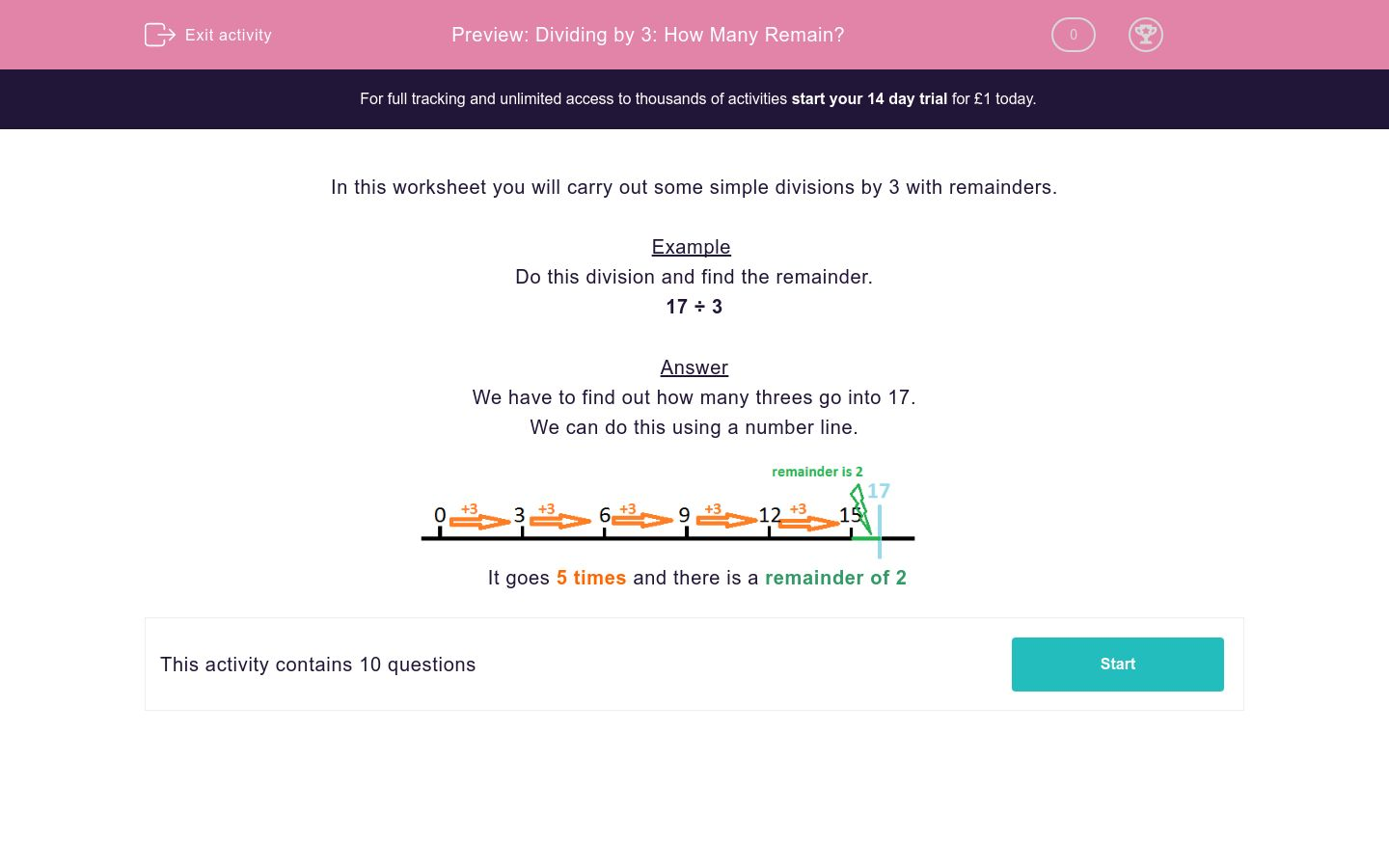# Dividing by 3: How Many Remain?

In this worksheet, students divide two-digit numbers by 3 and note down how many remain.Key stage:  KS 2

Curriculum topic:   Maths and Numerical Reasoning

Curriculum subtopic:   Mixed Problems

Difficulty level:### QUESTION 1 of 10

In this worksheet you will carry out some simple divisions by 3 with remainders.

Example

Do this division and find the remainder.

17 ÷ 3

We have to find out how many threes go into 17.

We can do this using a number line.It goes 5 times and there is a remainder of 2

---- OR ----